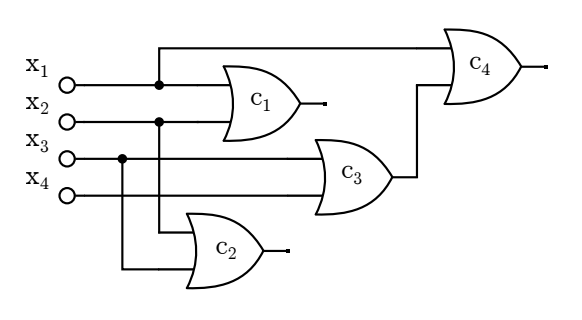시간 제한메모리 제한제출정답맞힌 사람정답 비율
4 초 1024 MB138763.636%

## 문제

Mirko is building a simple logic circuit in his workshop. The circuit consists of n starting wires denoted with x1, x2, . . . , xn and m logic elements OR denoted with c1, c2, . . . , cm. Each element has exactly two inputs and one output. Each of the inputs is connected to either a starting wire xj or to the output of another element cj. Of course, there are no cycles in a logic circuit and, moreover, it holds that the input of cj can be connected to the output of ci only when it holds i < j.

Each starting wire in the circuit can be set to value 0 or 1, and the value of the output of each element is the logic OR operation of its inputs — the value is 0 if the values of both inputs are 0, otherwise it is 1.

Mirko does not know the initial values of the starting wires, but with careful measurements, he has determined the values of the output of some elements. Find the remaining values of the outputs that can be unambiguously determined based on the measurements.

## 입력

The first line of input contains the positive integers n and m — the number of starting wires and the number of elements in the circuit. The following line contains a string of exactly m characters that describes the measured value of the output of the element cj, or is equal to “?” if Mirko did not perform this measurement. The j th of the following m lines contains labels of two inputs of the circuit cj, each label being either a label of the starting wire in the form of “xi” where it holds 1 ≤ i ≤ n, or a label of the element “ci” where it holds 1 ≤ i < j. The two inputs of the element cj may be the same. You can assume that the measured values are mutually consistent.

## 출력

The first line of output must contain a string of m characters — the j th character in the string must correspond to the value of the output of cj or be “?” if that value cannot be unambiguously determined.

## 서브태스크

번호배점제한
17

n ≤ 15, m ≤ 20

242

n ≤ 500, m ≤ 500

351

n ≤ 10 000, m ≤ 10 000

## 예제 입력 1

4 4
10??
x1 x2
x2 x3
x3 x4
x1 c3


## 예제 출력 1

10?1## 예제 입력 2

4 5
11???
x1 x2
x3 x4
x1 x3
x2 x4
c3 c4


## 예제 출력 2

11??1


## 채점 및 기타 정보

• 예제는 채점하지 않는다.